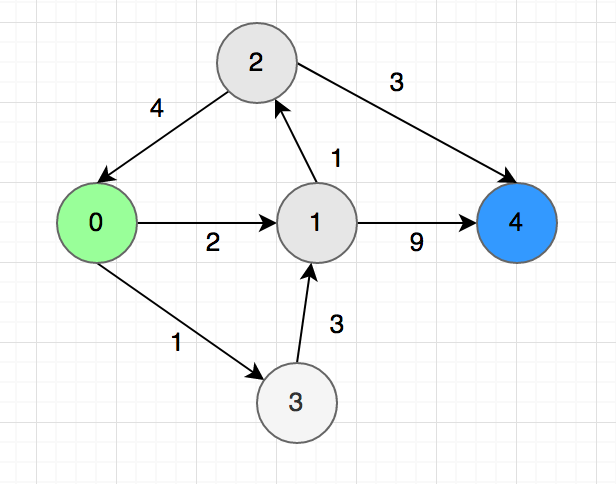# Dynamic Programming 2 (Shortest Path, DAG)

In the first part, we learned about the Fibonacci Number. In this article, we will talk about the shortest path problem and will learn some properties of Dynamic Programming along the way. The shortest path is a graph problem but you don’t really need to know about graph algorithms for this article.

Let’s say we need to find the shortest between one city to another. There are $n$ cities in total, $0$ is the first city and $n-1$ is the last. The path and distance between pairs of cities are marked with arrows like the picture below:Now we need to find the length of the shortest path between $0$ to $n-1$.

#### Finding Subproblems/States:

First, we need to find that are the parameters or states can define the problem. Remember the word ‘state’, we will be using it a lot. For this problem, the state is defined by the city you are currently in. Let’s say you are in city $u$, we will express our problem as a function $F(u)$. Just like Fibonacci, we will now try to find a recursive solution.

#### State Transition and Recursion

We don’t know which is next city to from $u$ to reach the destination in the shortest path. In this step, we will guess the next city and every guess will be a new subproblem. In the graph, we can go to the city $1$ and $3$ from $0$. So we will get the shortest path from $f(0)$ using this formula:

$f(0) = min(f(1) + 2, f(3) + 1)$

The idea is to go to each adjacent city and find the shortest path from there. The answer will bee the minimum of all the guesses. In general:

$f(n – 1) = 0 \\f(u) = min(f(u, v) + w(u, v)) \ where \ (u,v) \in E \ for\ u \ne n – 1$

Here $w(u, v)$ is the distance between $u$ and $v$.

#### Sub-problem Ordering

Now let’s think about the ordering of the function calls after we run the recursion.Now we are in big trouble, the subproblems created a cycle. For example, to find the value of $f(0), we need to. know the value of$f(0)$but$f(2) again depends of $f(0)$. If we try to run this as a code, it will stick in an infinite loop. This brings us to the concept of DAG.

#### Directed Acyclic Graph (DAG)

A DAG or directed acyclic graph is a directed graph with no cycles. A dynamic programming solution will work only if the subproblems create a DAG.

Even though we can’t solve the shortest path problem in a general graph using the formula above, we still can solve it for a DAG. In the graph above, we can remove the $2->0$ arrow and it will become DAG.

#### The C++ Code

Now we can solve it using DP. The C++ code will be like this:

One interesting fact is, we can still find the shortest path a general graph using DP but we need to add an extra parameter $k$ which indicates the maximum number of edges we are allowed to use. Rings a bell? Yes, that is very similar to the bellman ford algorithm.

#### Complexity

We have $n$ subproblems in this problem $f(0), f(1)…f(n-1)$. Moreover, for every city $u$, we are going to a maximum of $n$ adjacent cities. Total complexity will be the multiplication of the number of subproblems and the complexity of internal operations which is $O(n^2)$.

#### Iterative Version

For this problem, writing the iterative version is a bit difficult but possible. For the iterative version, we need to arrange the subproblems in topological order because, in each subproblem, you must make sure you solved the dependant subproblems. For Fibonacci, it was very easy but for this graph, it’s a bit tricky. You can google about topological sorting in a graph, I also discussed in brief here.

For the example above, we will get ${0, 3, 1, 2, 4}$ after sorting. Now you can loop this array in reversed order for building the table. It’s ok if you can’t implement that for this problem, it’s quite complicated and won’t give any advantage. We will learn more about the Iterative version when we learn LIS and knapsack.

#### Conclusion

So far you have learned:

• How to define the subproblems
• How to transit from one subproblem to another to find the optimal solution
• What is DAG, why DP can’t work if there is a cyclic dependency?

Still not getting confidence? No problem, this just the start, you will be able to solve some problems after you read the next part.

Happy Coding